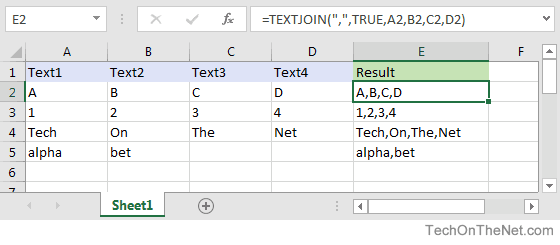# MS Excel: How to use the TEXTJOIN Function (WS)This Excel tutorial explains how to use the Excel TEXTJOIN function with syntax and examples.

## Description

The Microsoft Excel TEXTJOIN function allows you to join 2 or more strings together with each value separated by a delimiter.

The TEXTJOIN function is a built-in function in Excel that is categorized as a String/Text Function. It can be used as a worksheet function (WS) in Excel. As a worksheet function, the TEXTJOIN function can be entered as part of a formula in a cell of a worksheet.## Syntax

The syntax for the TEXTJOIN function in Microsoft Excel is:

`TEXTJOIN( delimiter, ignore_empty, text1, [ text2, ... text_n ] )`

### Parameters or Arguments

delimiter
A string inserted between each text value in the resulting string. Most commonly, you would use a delimiter such as a comma or space character.
ignore_empty
Determines whether empty values are included in the resulting string. TRUE ignores empty values and FALSE includes empty values in the result.
text1, text2, … text_n
The strings that you wish to join together. There can be up to 252 strings that are joined together.

• Excel 2016

## Type of Function

• Worksheet function (WS)

## Example (as Worksheet Function)

Let’s look at some Excel TEXTJOIN function examples and explore how to use the TEXTJOIN function as a worksheet function in Microsoft Excel:Based on the Excel spreadsheet above, the following TEXTJOIN examples would return:

```=TEXTJOIN(",",TRUE,A2,B2,C2,D2)
Result: "A,B,C,D"

=TEXTJOIN(",",TRUE,A3,B3,C3,D3)
Result: "1,2,3,4"

=TEXTJOIN(",",TRUE,A4,B4,C4,D4)
Result: "Tech,On,The,Net"

=TEXTJOIN(",",TRUE,A5,B5,C5,D5)
Result: "alpha,bet"

=TEXTJOIN(" ",TRUE,A4,B4,C4,D4,"is","great")
Result: "Tech On The Net is great"```